# Make an Arduino Temperature Sensor (thermistor tutorial)

Thermistors are simple, inexpensive, and accurate components that make it easy to get temperature data for your projects.

BeginnerFull instructions provided5 hours37,354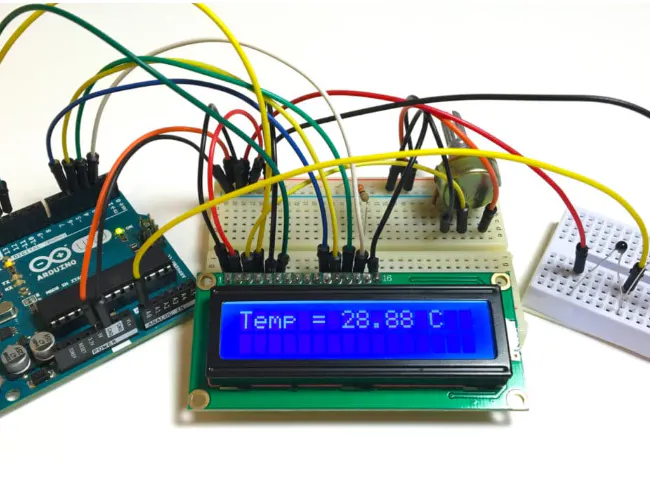## Things used in this project

### Hardware componentsArduino UNO
×1Jumper wires (generic)
×1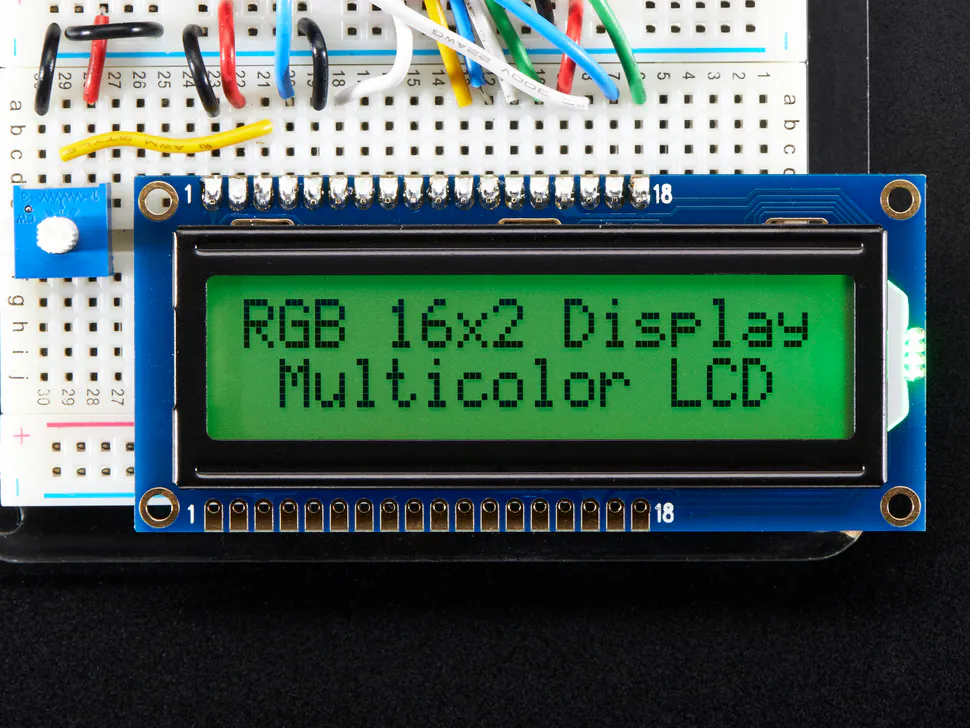Adafruit RGB Backlight LCD - 16x2
×1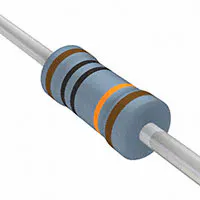Resistor 100k ohm
×1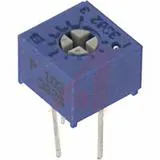Single Turn Potentiometer- 100k ohms
×1

### Software apps and online servicesArduino IDE
 www. Fritzing.org

## Custom parts and enclosures

### CONNECT THE CIRCUIT

Connect the thermistor and resistor to your Arduino like this: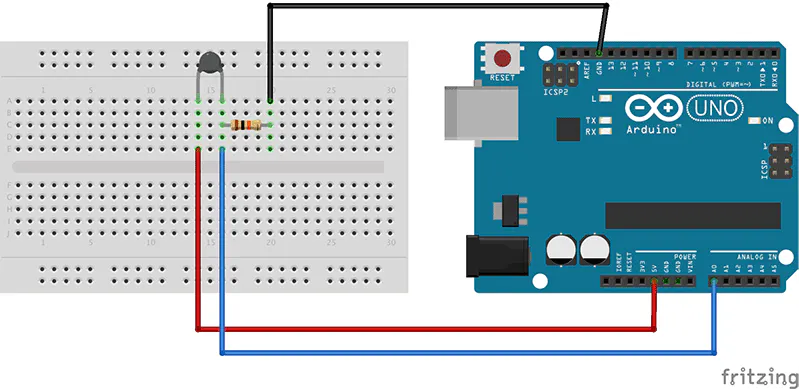## Schematics

### A BASIC THERMISTOR CIRCUIT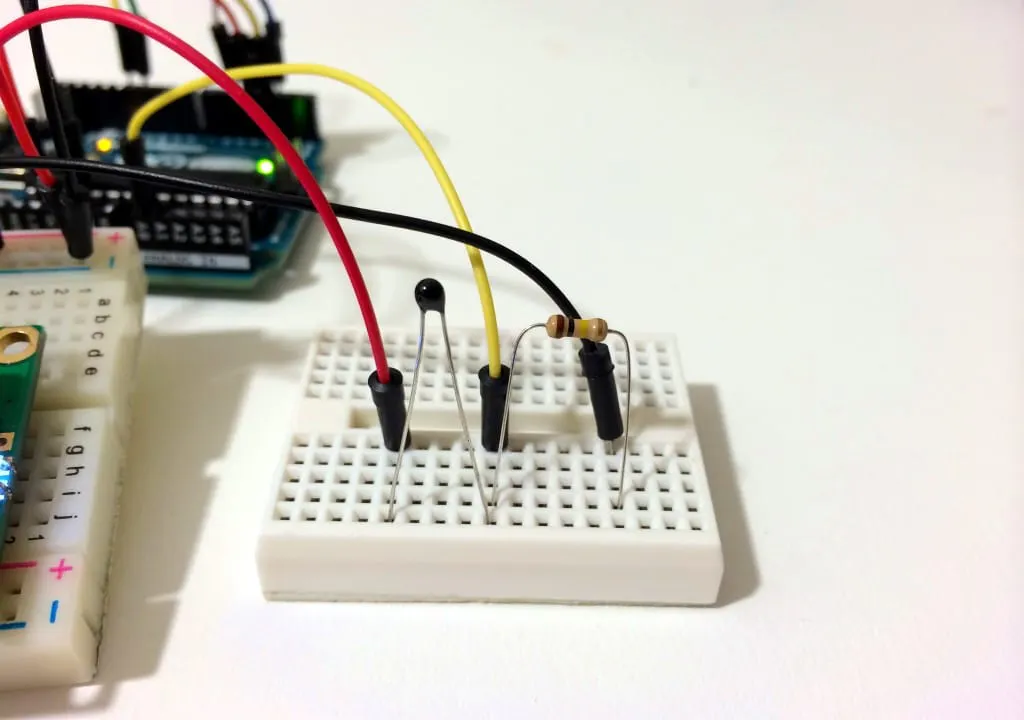## Code

### CODE FOR SERIAL MONITOR OUTPUT OF TEMPERATURE READINGS:

C/C++
After connecting the circuit above, upload this code to your Arduino to output the temperature readings to the serial monitor in Fahrenheit:
```int ThermistorPin = 0;
int Vo;
float R1 = 10000;
float logR2, R2, T;
float c1 = 1.009249522e-03, c2 = 2.378405444e-04, c3 = 2.019202697e-07;

void setup() {
Serial.begin(9600);
}

void loop() {

R2 = R1 * (1023.0 / (float)Vo - 1.0);
logR2 = log(R2);
T = (1.0 / (c1 + c2*logR2 + c3*logR2*logR2*logR2));
T = T - 273.15;
T = (T * 9.0)/ 5.0 + 32.0;

Serial.print("Temperature: ");
Serial.print(T);
Serial.println(" F");

delay(500);
}
```

### CODE FOR LCD OUTPUT OF TEMPERATURE READINGS

C/C++
To output the temperature readings to a 16X2 LCD.
```#include <LiquidCrystal.h>

int ThermistorPin = 0;
int Vo;
float R1 = 10000;
float logR2, R2, T;
float c1 = 1.009249522e-03, c2 = 2.378405444e-04, c3 = 2.019202697e-07;

LiquidCrystal lcd(12, 11, 5, 4, 3, 2);

void setup() {
Serial.begin(9600);
}

void loop() {

R2 = R1 * (1023.0 / (float)Vo - 1.0);
logR2 = log(R2);
T = (1.0 / (c1 + c2*logR2 + c3*logR2*logR2*logR2));
T = T - 273.15;
T = (T * 9.0)/ 5.0 + 32.0;

lcd.print("Temp = ");
lcd.print(T);
lcd.print(" F");

delay(500);
lcd.clear();
}
```

### CODE FOR CELCIUS AND FAHRENHEIT

C/C++
This program will display Celsius and Fahrenheit at the same time:
```int ThermistorPin = 0;
int Vo;
float R1 = 10000;
float logR2, R2, T, Tc, Tf;
float c1 = 1.009249522e-03, c2 = 2.378405444e-04, c3 = 2.019202697e-07;

void setup() {
Serial.begin(9600);
}

void loop() {

R2 = R1 * (1023.0 / (float)Vo - 1.0);
logR2 = log(R2);
T = (1.0 / (c1 + c2*logR2 + c3*logR2*logR2*logR2));
Tc = T - 273.15;
Tf = (Tc * 9.0)/ 5.0 + 32.0;

Serial.print("Temperature: ");
Serial.print(Tf);
Serial.print(" F; ");
Serial.print(Tc);
Serial.println(" C");

delay(500);
}
```

## Credits

### Iasonas Christoulakis

13 projects • 7 followers